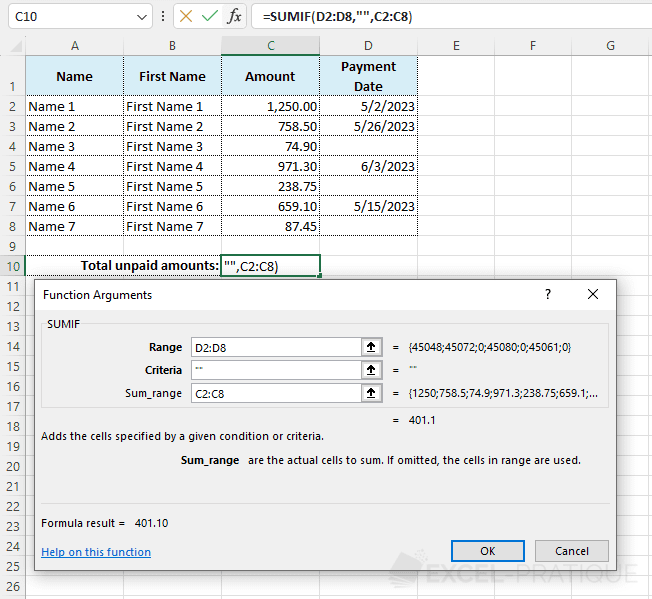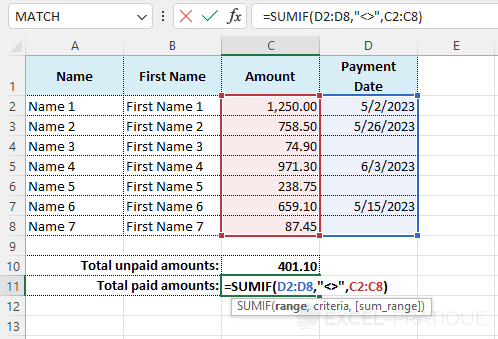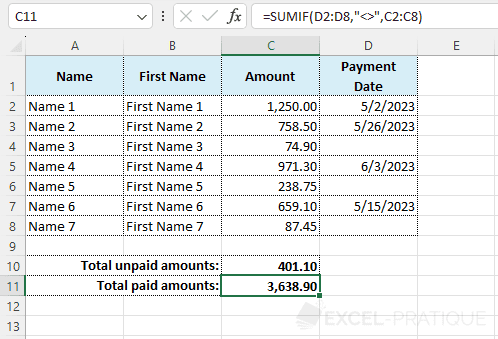# Excel function SUMIF

The SUMIF function makes it possible to calculate a sum taking specified conditions into account.

Here is an example:

We want to display the totals brought in by men and women separately (see image below).

To do this, click on C9 and select the SUMIF function.

• In the "Criteria" field, enter the criteria "Mr".
• In the "Range" field, enter the data range for which the criteria will be verified.
• In the "Sum_range" field, enter the data range which will be used to calculate the sum.

Click OK.

In this case, Excel will check to see if cells A1 through A8 contain the "Mr" specified in our criteria.

If this is actually the case, Excel will include the total associated with cells C1 through C8 in the final sum.

Example: A1 does not contain the criteria, so C1 will not be included in the sum. A2 corresponds to the criteria, so C2 will be included in the sum.Now we have calculated the total amount brought in by the men.

We can do just the same for the women by replacing "Mr" with "Ms".Here is the result as returned by the function: